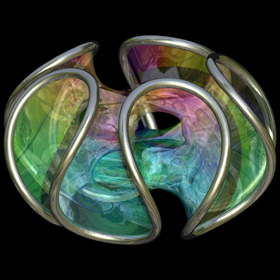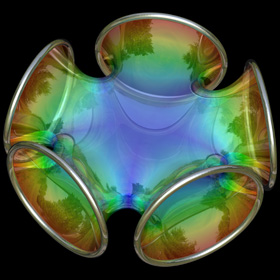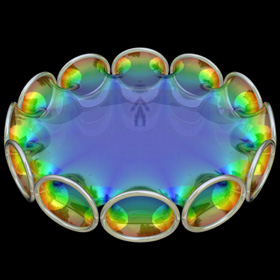## Archive for the 'Math' Category

25
Feb
09

### Chen-Gackstatter Minimal SurfaceThe Chen-Gackstatter Minimal Surface is a modified Enneper surface with holes in it. The following Mathematica code uses some functions that were adapted from Matthias Weber’sMathematica notebook:
```(* runtime: 0.4 second *) << Graphics`Shapes`; k = 5; n = (k - 1)/k; rho = 1.0/Sqrt[4^n Gamma[(3 - n)/2] Gamma[1 + n/2]/(Gamma[(3 +n)/2]Gamma[1 - n/2])]; phi[n_, z_] := z^(1 + n)Hypergeometric2F1[(1 + n)/2, n, (3 + n)/2, z^2]/(1 + n); f[z_] := {0.5(phi[n, z]/rho - rho phi[-n, z]), 0.5I(rho phi[-n, z] + phi[n, z]/rho), z}; surface = ParametricPlot3D[Re[f[r Exp[I theta]]], {r, 0, 2}, {theta, 1*^-6, 2Pi}, PlotPoints -> {9, 33}, Compiled -> False, DisplayFunction -> Identity][]; Show[Graphics3D[Table[RotateShape[surface, 0, 0, 2Pi i/k], {i, 0, k - 1}]]]```

Advertisements
10
Feb
09

### Scherk-Collins SurfaceThis surface can be formed by twisting and warping a singly-periodic Scherk’s minimal surface. This idea was originally attributed to Brent Collins. Technically, the surface is no longer considered exactly “minimal” after twisting but it still looks minimal (it is actually very difficult to find the exact shape for most minimal surfaces). Click here to download some POV-Ray code.Here is some Mathematica code:
```(* runtime: 0.3 second *) << Graphics`Master`; n = 5; r = 0.75n; Twist[{x_, y_, z_}, theta_] := {x Cos[theta] - y Sin[theta], x Sin[theta] + y Cos[theta], z}; Warp[{x_, y_, z_}, theta_] := {(x + r) Cos[theta], (x + r) Sin[theta], y}; f[z_] := Module[{t1 = Sqrt[2Cot[z]], t2 = Cot[z] + 1}, Warp[Twist[Re[{0.5xsign(Log[t1 - t2] - Log[t1 + t2])/Sqrt, ysign I(ArcTan[1 - t1] - ArcTan[1 + t1])/Sqrt, z}], 2Re[z]/n], 2Re[z]/n]]; DisplayTogether[Table[ParametricPlot3D[f[x + I y], {x, 0, n Pi}, {y, 0.001, 0.75}, PlotPoints -> {8n + 1, 5}, Compiled -> False], {xsign, -1, 1, 2}, {ysign, -1, 1, 2}]]```

The following Mathematica code can be used to increase the number of edges (or “branches”). This code uses some complicated functions that were adapted from Matthias Weber’s Mathematica notebook:
```(* runtime: 1.2 seconds *) << Graphics`Shapes`; k = 4; phi = Pi(0.6/k - 0.5)/(1 - k); f[z_] := Re[NIntegrate[Evaluate[{0.5 (w^(1 - k) - w^(k - 1)), 0.5 I (w^(1 - k) + w^(k - 1)), 1}/(w^(k + 1) + w^(1 - k) - 2w Cos[k phi])], {w, 0, z}]]; alpha = Pi/k; zbeta = Exp[I Pi(phi/alpha - 0.5)]; surface = ParametricPlot3D[Re[f[Exp[I alpha/2]((1 + I zbeta Exp[r + I theta])/(I Exp[r + I theta] -zbeta))^(alpha/Pi)]], {r, 0, 4}, {theta, 0, Pi}, PlotPoints -> 10, Compiled -> False, DisplayFunction -> Identity][]; z0 = f[]; surface = {surface, AffineShape[TranslateShape[surface, {0, 0, -2z0}], {1, 1, -1}]}; surface = {surface, AffineShape[surface, {1, -1, 1}]}; surface = Table[RotateShape[surface, 2Pi i/k, 0, 0], {i, 1, k}]; dz = Pi Csc[k phi]/k; Show[Graphics3D[Table[TranslateShape[surface, {0, 0, i dz}], {i, 0, 1}]]] ```

21
Jan
09

### Punctured HelicoidHere is a helicoidwith holes in it. The following Mathematica code uses some complicated functions that were adapted from Matthias Weber’sMathematica notebook:
```(* runtime: 4 seconds *) << Graphics`Shapes`; tau0 = Exp[1.23409 I]; b0 = 0.629065; theta[z_] := EllipticTheta[1, Pi z, Exp[I Pi tau0]]; r1[z_] := theta[z + 0.5 (b0 - 2) (tau0 + 1)]/theta[z + 0.5 (b0 - 1) (tau0 + 1)]; r2[z_] := theta[z - 0.5 b0 (tau0 + 1)]/theta[z - 0.5 (b0 + 1) (tau0 + 1)]; omega3[z_] := r1[z] r2[z]/(0.386191 - 0.169839 I); G[z_] := (108.37 - 62.8417 I) Exp[I Pi (b0 - 2 z + 2 tau0 + b0 tau0)]r1[z]/r2[z]; f[z0_] := Re[NIntegrate[Evaluate[{-(G[z] omega3[z] - omega3[z]/G[z] )/2, I(G[z] omega3[z] + omega3[z]/G[z] )/2, omega3[z]}], {z, tau0/2, z0}]] + {0.434156, 0, -1}; a0 = -0.409956; r0 = 2.43051; g[z_] := (EllipticF[ArcSin[(a0 + r0 E^z)/(1 - a0 E^z)], 1/r0^2]/(2EllipticF[Pi/2, 1/r0^2]) + 0.5)(1 + tau0)/2; surface = ParametricPlot3D[f[g[x + I y]], {x, -2.5, 2.5 - 0.8881}, {y, 0.001,0.999Pi}, PlotPoints -> {15, 10}, Compiled -> False, DisplayFunction -> Identity][]; surface = {surface, RotateShape[surface, 0, 0, Pi]}; Show[Graphics3D[{surface, TranslateShape[surface, {0, 0, 2}]}, ViewPoint -> {1, 6, 3}]];```

16
Jan
09

### Jorge-Meeks K-NoidsThe following Mathematica code uses some functions that were adapted from Matthias Weber’sMathematica notebook:
```(* runtime: 0.4 second *) << Graphics`Shapes`; k = 5; phi1[z_] := z^(k - 1) (k/(1 - z^k) - (k - 1) LerchPhi[z^k, 1, 1 - 1/k])/k^2; phi2[z_] := z(1/(1 - z^k) + (k - 1)LerchPhi[z^k, 1, 1/k]/k)/k; f[z_] := {0.5 (phi2[z] - phi1[z]), 0.5 I (phi1[z] + phi2[z]), 1/(k - k z^k)}; surface = ParametricPlot3D[Re[f[(1 + 2/(I Exp[x + I y] - 1))^(2/k)]], {x,0, Pi/2}, {y, -Pi/2, Pi/2}, PlotPoints -> {8, 16}, Compiled -> False, DisplayFunction -> Identity][]; surface = {surface, AffineShape[surface, {1, -1, 1}]}; Show[Graphics3D[Table[RotateShape[surface, 0, 0, 2Pi i/k], {i, 0, k - 1}]]];```

08
Jan
09

### Catenoid/HelicoidThis minimal surface is a cross between acatenoid andhelicoid. It would be interesting to see what really happens when a spring is covered with a soap film. Click here to download some POV-Ray code. Here is some Mathematica code:
```(* runtime: 0.6 second *) x := Sin[alpha]Cosh[v]; y := Cos[alpha]Sinh[v]; Do[ParametricPlot3D[{x Cos[u] + y Sin[u], x Sin[u] - y Cos[u], u Cos[alpha] + v Sin[alpha]}, {u, 0, 2Pi}, {v, -2.25, 2.25}, PlotPoints -> {36, 10}], {alpha, -Pi/2, Pi/2, Pi/18}];```

21
Oct
08

### Dodecaplex (120 Cell)Polychorons are the 4D version of polyhedrons. One way to visualize a polychoron is to apply a 4D to 3D stereographic projection to it. A dodecaplex is a uniform 4D polychoron composed 120 dodecahedral cells. These cells can be divided into 12 rings (Hopf fibrations) of 10 cells each. This picture shows a stereographic projection of 6 rings of the dodecaplex. Each ring is shown in a different color, but only 5 rings are open to direct view because they are wrapped around the 6th ring. I first saw this concept on Matthias Weber’s book page. Click here to download some POV-Ray code.10
Oct
08

### Stereographic Projection of a DodecahedronHere is a stereographic projection of a dodecahedron. This is the 3D counterpart to the 4D dodecaplex. Here is some Mathematica code:
```(* runtime: 0.4 second *) z1 = (Sqrt - 3)/Sqrt[30.0 - 6 Sqrt]; z2 = Sqrt[(1 + 2/Sqrt)/3.0]; r1 = Sqrt[2(1 + 1/Sqrt)/3.0]; r2 = Sqrt[2(1 - 1/Sqrt)/3.0]; vertices = Join[Table[{r2 Cos[theta], r2 Sin[theta], z2}, {theta, 0, 2Pi - 0.4Pi, 0.4Pi}], Table[z1 = -z1; {r1 Cos[theta], r1 Sin[theta], z1}, {theta, 0, 1.8Pi, 0.2Pi}], Table[{r2 Cos[theta], r2 Sin[theta], -z2}, {theta, 0.2Pi, 1.8Pi, 0.4Pi}]]; edges = {{1, 2}, {2, 3}, {3, 4}, {4, 5}, {5, 1}, {1, 6}, {2, 8}, {3, 10}, {4, 12}, {5, 14}, {6, 7}, {7, 8}, {8, 9}, {9, 10}, {10, 11}, {11, 12}, {12, 13}, {13, 14}, {14, 15}, {15, 6}, {7, 16}, {9, 17}, {11, 18}, {13, 19}, {15, 20}, {16,17}, {17, 18}, {18, 19}, {19, 20}, {20, 16}}; Show[Graphics3D[Map[Line[vertices[[#]]] &, edges]]] Norm[x_] := x.x; Normalize[x_] := x/Sqrt[x.x]; Rx[theta_] := {{1, 0, 0}, {0, Cos[theta], -Sin[theta]}, {0,Sin[theta], Cos[theta]}}; ProjectPoint[{x_, y_, z_}] := 2{x, y}/(1 - z); ProjectSegment[{v1_, v2_}] := Module[{p1 = ProjectPoint[v1], p2 = ProjectPoint[v2]}, {nx, ny, nz} = Normalize[Cross[v1, v2]]; If[nz != 0, p0 = -2{nx, ny}/nz; r = 2/Abs[nz]; theta = Sign[nz]Re[ArcCos[(p1 - p0).(p2 - p0)/Sqrt[Norm[p1 - p0]Norm[p2 - p0]]]], theta = 0]; If[Abs[theta] > 0.001, theta1 = ArcTan[p1[] - p0[], p1[] - p0[]]; theta2 = theta1 + theta; If[theta1 > theta2, t = theta1; theta1 = theta2; theta2 = t]; Circle[p0, r, {theta1, theta2}], Line[{p1, p2}]]]; Do[Show[Graphics[Map[ProjectSegment[Map[Rx[phi].# &, vertices[[#]]]] &, edges], PlotRange -> 6{{-1, 1}, {-1, 1}}, AspectRatio -> Automatic]], {phi, 0, 2Pi, Pi/18}];```

## Welcome !

You will find here some of my favorite hobbies and interests, especially science and art.

I hope you enjoy it!

Subscribe to theRSS feed to stay informed when I publish something new here.

I would love to hear from you! Please feel free to send me an email : bugman123-at-gmail-dot-com

## Recent CommentsBerna Blalack on Magnetic Pendulum Strange…Daan on Magnetic Pendulum Strange…Sebastian Schepis on Diffusion Limited Aggregation…mohammad_andito on CFM56-5 Turbofan Jet Engi…SasQ on Magnetic Field of a Solen… OUPblog » Blog… on Diamond Light Dispersion Complex Roots on Polynomial Roots Joukowski airfoils |… on Joukowski AirfoilKarim Alame on Flapping WingREJISH J on Joukowski Airfoil SOLINOID | Materials… on Magnetic Field of a Solen…Emanuele on 4D “Squarry” Julia…Emanuele on Hydrogen Electron Orbital Prob…Tim on Mandelbrot Set Pickover S…khankasi1 on 4D Quaternion Mandelbrot …

• 561,072 hits
Advertisements